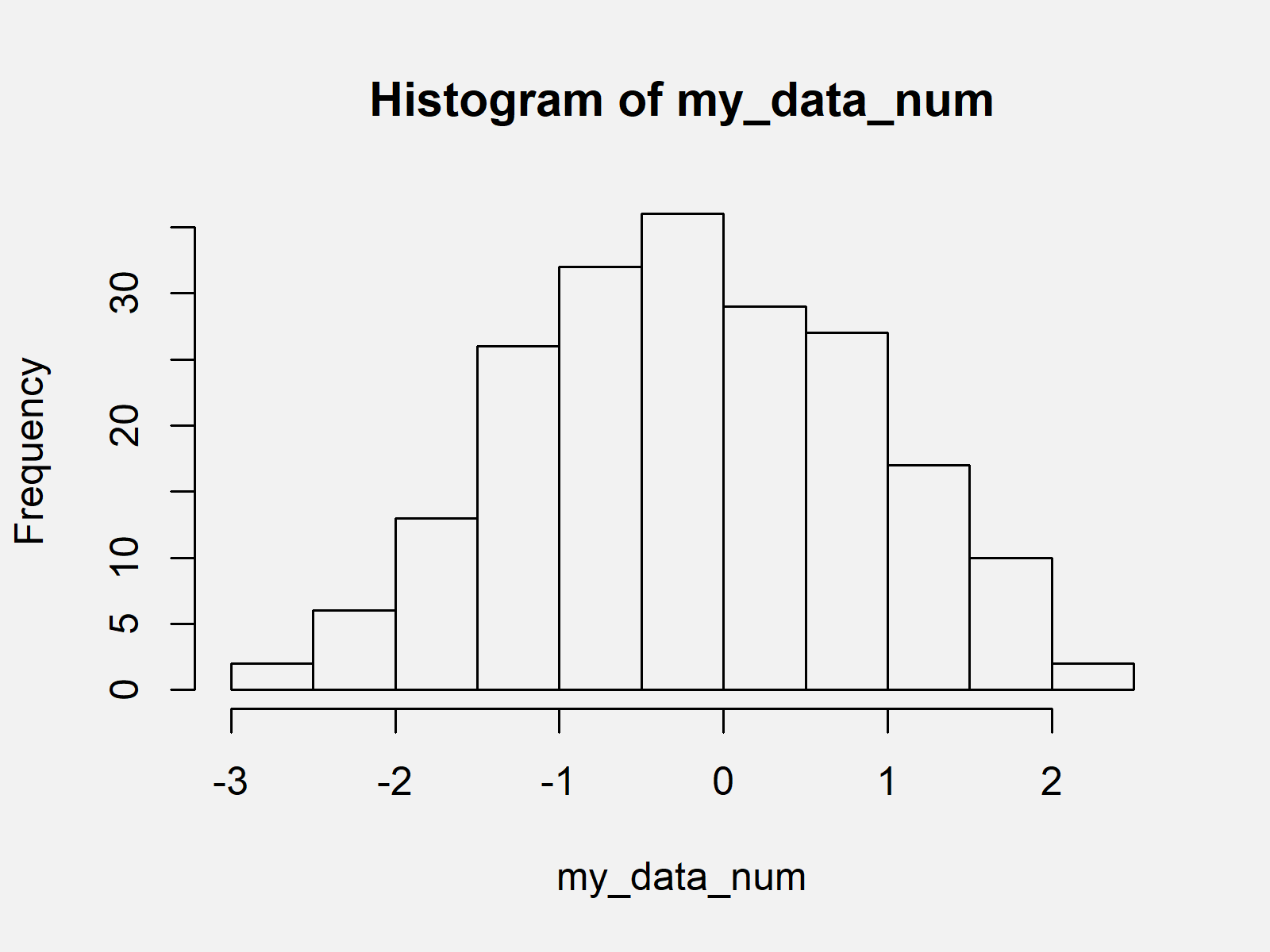# R Error in hist.default : ‘x’ must be numeric (2 Examples)

In this tutorial, I’ll illustrate how to handle the error in hist.default(X) : ‘x’ must be numeric in R.

The tutorial will contain two examples for the error message “x must be numeric”. To be more specific, the article will contain these content blocks:

Let’s take a look at some R codes in action…

## Creation of Example Data

We’ll use the following data as basement for this R tutorial:

```set.seed(64982057) # Create example data my_data <- as.character(round(rnorm(200), 2)) my_data # Print first values of data #  "-0.1" "0.65" "-0.71" "-0.55" "-1.93" "1.69" "-0.91" "-1.02" "0.28" "0.3" ...```

The previous output of the RStudio console shows the structure of our exemplifying data – It’s a vector of random numbers.

Let’s assume that we want to draw these random numbers in a histogram…

## Example 1: Reproduce the Error in hist.default(X) : ‘x’ must be numeric

In this example, I’ll illustrate how to replicate the error message in hist.default(X) : ‘x’ must be numeric.

Consider that we want to apply the hist function to our random data:

```hist(my_data) # Trying to create histogram # Error in hist.default(my_data) : 'x' must be numeric```

As you can see, the previous R code returned the error in hist.default(my_data) : ‘x’ must be numeric.

The reason for this is that our input data is not numeric. We can check that by using the class function:

```class(my_data) # Checking class of data #  "character"```

Our example data object is a character vector. However, the hist function only takes numeric data as input.

Let’s solve this problem…

## Example 2: Fix the Error in hist.default(X) : ‘x’ must be numeric

Example 2 shows how to get rid of the error in hist.default(X) : ‘x’ must be numeric. For this, we have to convert our data from character to numeric:

```my_data_num <- as.numeric(my_data) # Convert character to numeric my_data_num # Print first values of data #  -0.10 0.65 -0.71 -0.55 -1.93 1.69 -0.91 -1.02 0.28 0.30 ...```

Now, we can apply the hist function to our updated data:

`hist(my_data_num) # Draw histogram in R`Figure 1 shows the output of the previous R programming syntax: A histogram created by the hist function of Base R.

## Video, Further Resources & Summary

Have a look at the following video of my YouTube channel. In the video, I’m illustrating the contents of this article.

Furthermore, you might read the related tutorials on my website.

In this R post you learned how to deal with the error in hist.default(X) : ‘x’ must be numeric. Please note that this error message can also occur when using other functions that need numeric values as input such as the cor() function when calculating correlations.